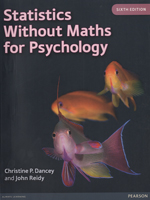Statistics Without Maths for Psychology, 6th editionBy: Chrstine P. Dancey & John Reidy September 2014 Pearson Education Distributed by Trans-Atlantic Publications Inc. ISBN: 9780273774990 612 Pages, Illustrated \$97.50 Paper Original Description: For students who need to understand and use statistics but find the mathematical formulae daunting,Statistics without Maths for Psychology is the ideal guide. The clear, straightforward style and step-by-step SPSS walkthroughs take you through all the statistical procedures you will need. Activities and questions enable you to test your learning and increase your understanding in a practical, manageable way. 1 Variables and research design 2 Introduction to SPSS 3 Descriptive statistics 4 Probability, sampling and distributions 5 Hypothesis testing and statistical significance 6 Correlational analysis: Pearson's r 7 Analyses of differences between two conditions: the t-test 8 Issues of significance 9 Measures of association 10 Analysis of differences between three or more conditions 11 Analysis of variance with more than one IV 12 Regression analysis 13 Analysis of three or more groups partialling out effects of a covariate 14 Introduction to factor analysis 15 Introduction to multivariate analysis of variance (MANOVA) 16 Non-parametric statistics Appendix 1: Table of z-scores and the proportion of the standard normal distribution falling above and below each score